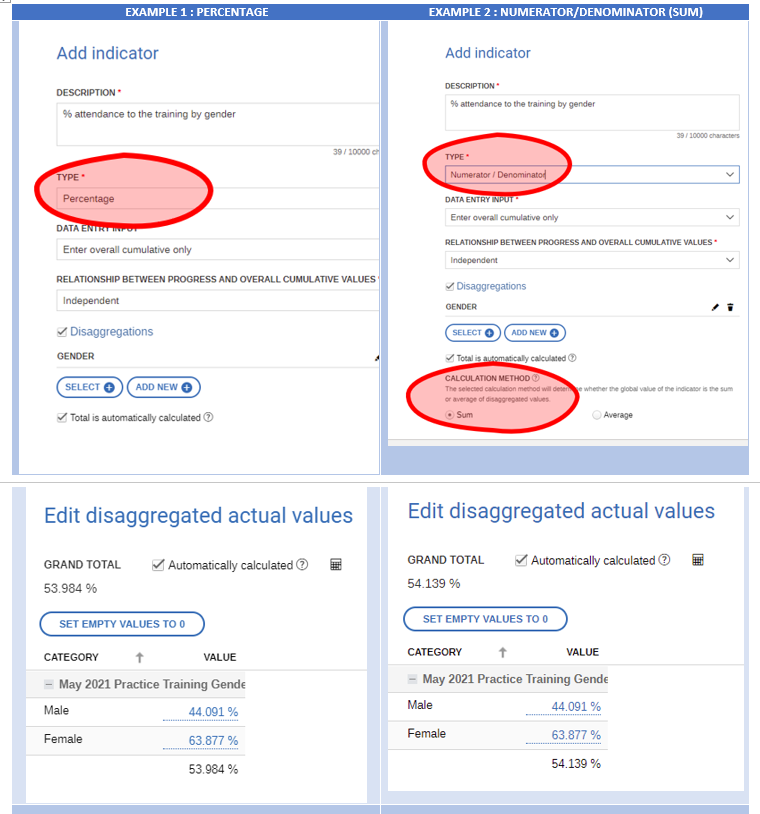# Numerator denominator VS Percentage indicators

This article explains the difference between selecting Numerator/ Denominator Indicator VS selecting a Percentage indicator

### Example

You have a group of 447 participants. Of these participants, 220 are male, and 227 are female.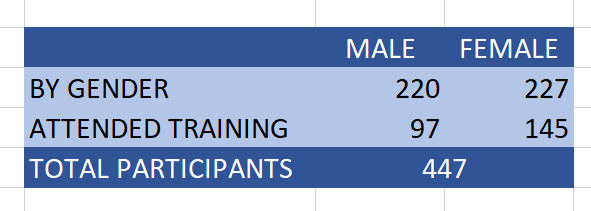Lets say we need to calculate the % of participants who fulfill a condition, disaggregated by gender, let's say the condition is "Attended training".

### Option 1: Using "Percentage" Indicator

In this case, LogAlto takes the overall values of the people who fulfill the condition and divide it among the number of disaggregations.

Using the example presented above, if 145 female participants and 97 male participants attended the training, this is what LogAlto calculates: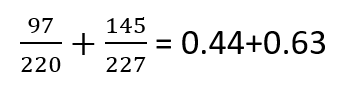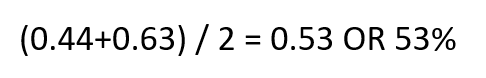This calculation doesn’t take into consideration the “weight” of each option. It simply counts the number of options and divides by that amount

### Option 2: Using Numerator/Denominator as the type of indicator

In this case, the system will "weigh" the options to reflect in the total amount the size of the group.

Using the example presented above, LogAlto would calculate this: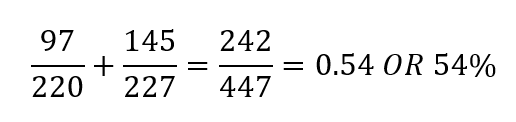This calculation takes into consideration the “weight” of each option, the final total is calculated based on this.

### Comparing the two options

As you can see, the results in both cases are slightly different. This is because in Option 1, we are taking the totals and dividing them by the number of disaggregations, whereas in Option 2 we are weighing the values to the size of the group.

Option 2 usually gives a more accurate result.